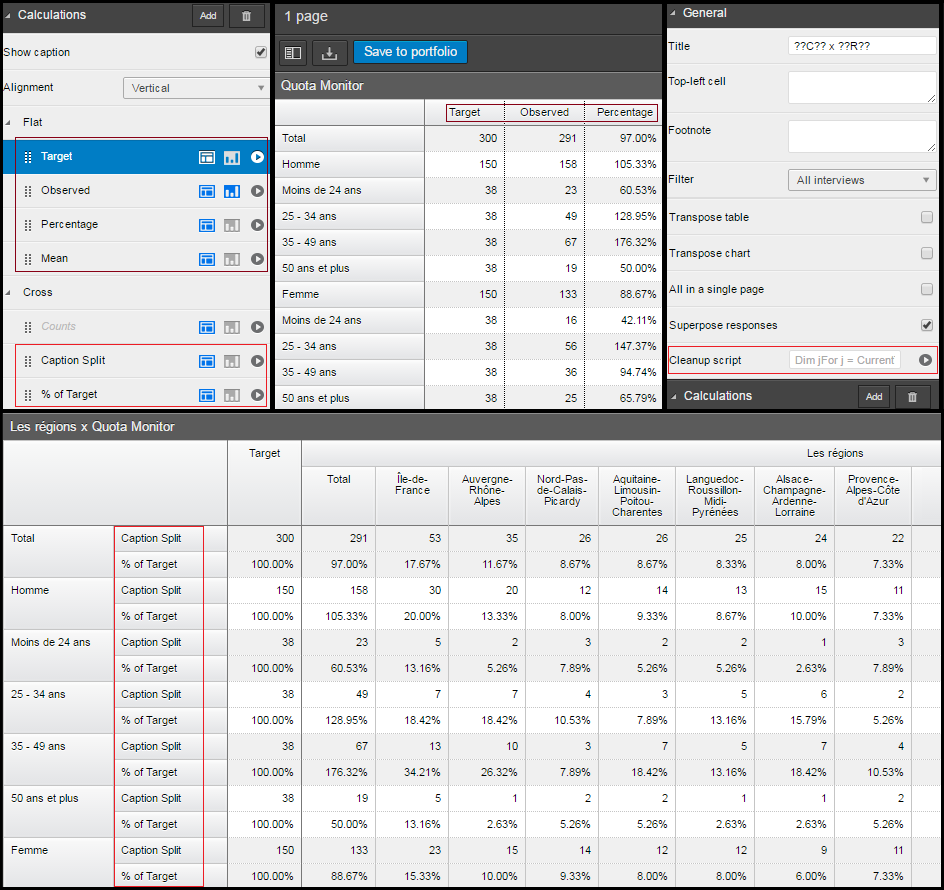Scroll

# Quota Monitoring for Analyse and Vista

Follow
 Summary This article shows the use of the 'read quota' function in a 'Closed by scripts' created variable. We combine this function with table arithmetic scripts to create a portfolio that will allow you to monitor the progress of your quotas as new data is collected. The examples are shown in Analyse and Vista 6 Applies to Askia Analyse, Askia Vista. Written for Researchers, Developers, Data Processors, Project Managers. Keywords Table arithmetic, Forloops, Coordinates, Data, Calculation arithmetic, Caption, Filtered, Quota, Split, Delimiter, Cleaning script, Closed by scripts, Cca, Supervisor, Connection string, Surveys, Server, Target, Replace, Text, Observed, Target, Array.

The .qes file and portfolio containing the example discussed is attached: Table Arithmetic Examples 13.rar.

Using table arithmetic we can create a portfolio in Analyse and Vista that will tell us what percentage of targets have been met for our quotas.

In this article I will show a few examples of how this can be done. In order to ensure all functionality required for these examples works, you will need to use Analyse version 5.3.5.2 from 13/04/2016 onwards and the latest version of Vista 6.0.3.8 (preferably 6.0.3.9).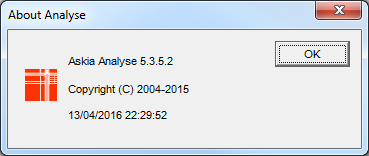The Quota Variable

The starting point for accomplishing each of the examples is to create a variable (Closed by scripts) based on your quota question. Right-click in the question tree > Create a variable… > Closed by scripts > hit the target button to the right of the ‘Script’ option: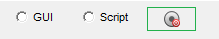This will bring up the following window: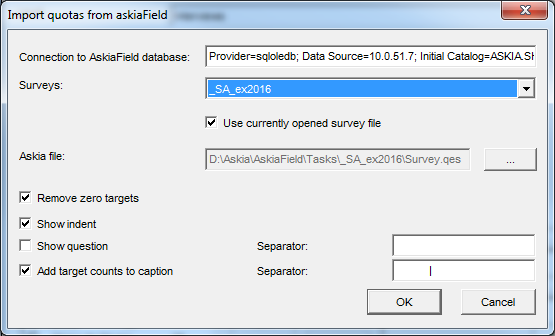The connection to the AskiaField database will be of this format:

And might look something like this:

• Provider=sqloledb; Data Source=10.0.10.1; Initial Catalog=YOUR.SERVER.Cca;Trusted_Connection=no;User Id=YOURID;YOURPASSWORD

In my case this is the Surveys database connection string from Supervisor > CCA settings > Tasks > Surveys >  with "Surveys" changed to "Cca" in the string

You may need to consult with your IT department or server admin on this point to get access.

This will automatically create your definitions and captions which include the quota target after your declared separator (in this case: ‘           |’).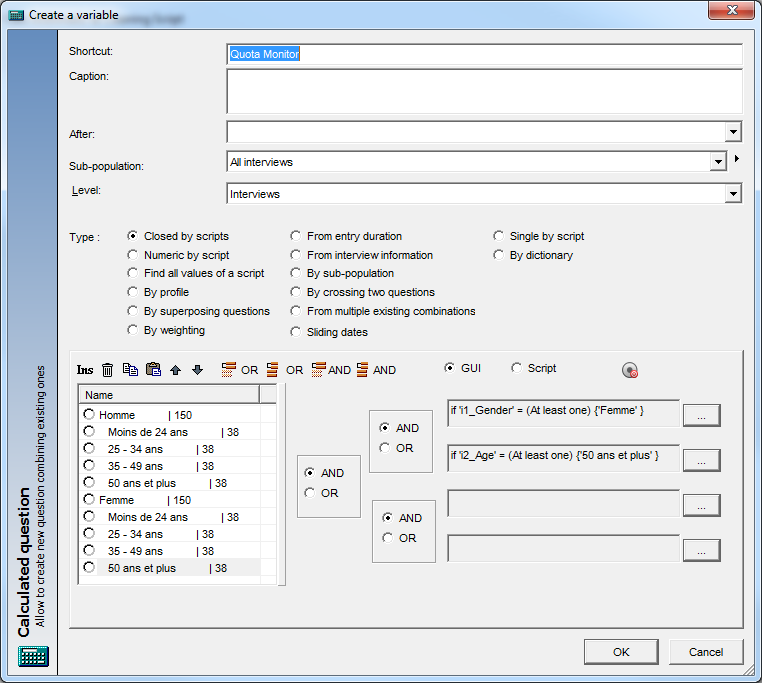Here is the quota set-up that is the source for this variable: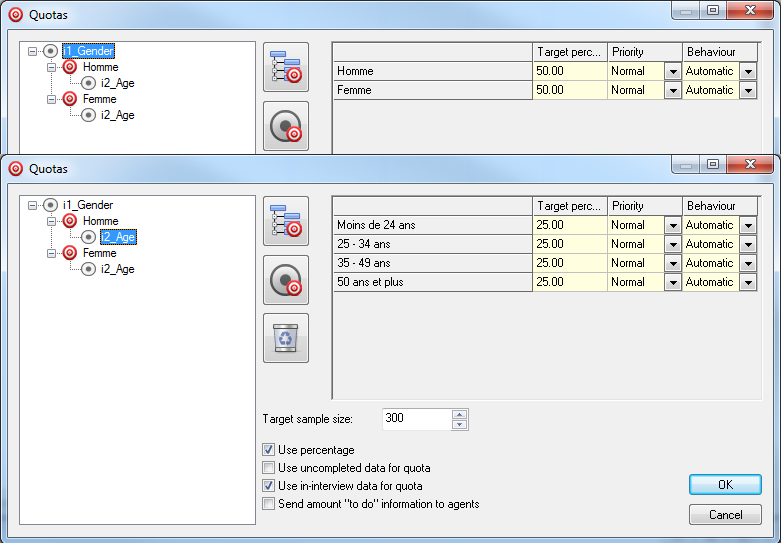Now the trick is to create a table with this variable and calculate the % of target achieved.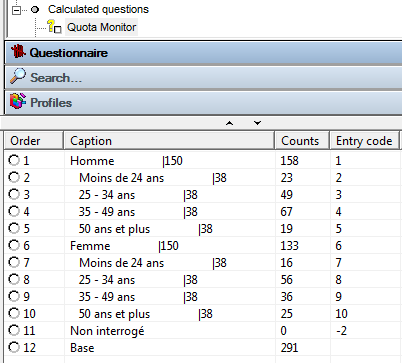e.g.  for code 5 (50 ans et plus) within (Homme) we have achieved 19 out of 38, so our % of target achieved will be 19 / 38 => 50%.

In the example portfolio you will see a number of tab definitions.

Example 1

The first example uses ‘[Ex1] Flat / Cleaning Script Only’ – It’s for a flat table (i.e. it is not broken by any column or edge)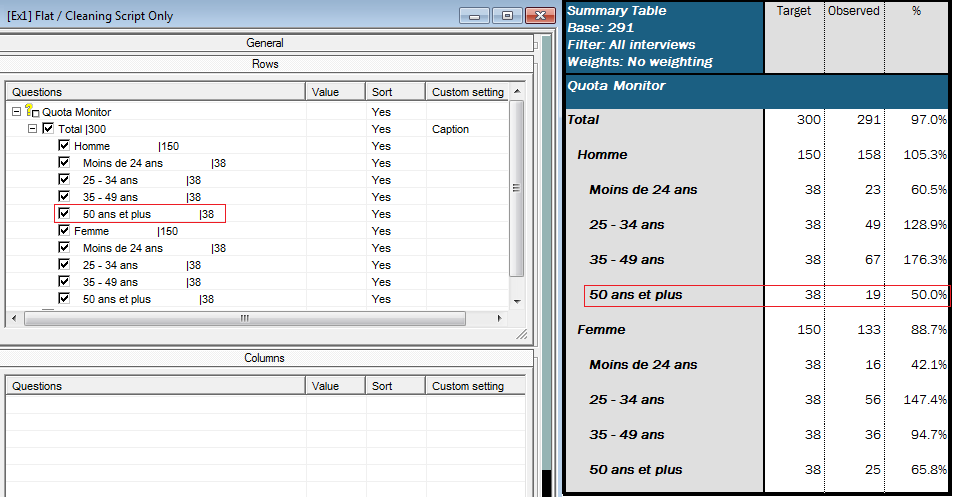The caption split and calculation are managed only by a cleaning script. Found in: General Tab > Settings > Sorting > Cleaning script.

Here is the table without the cleaning script: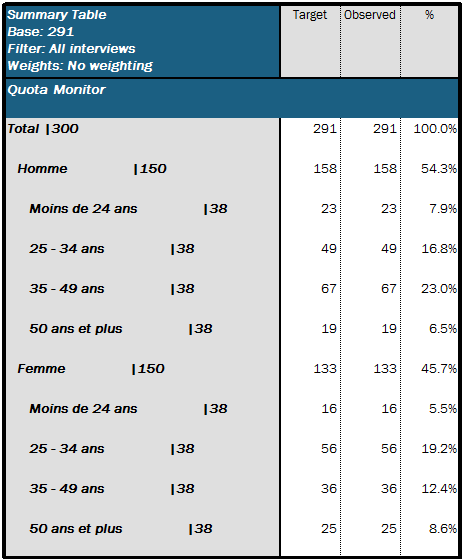The script below overwrites columns 1, 2 and 4 in the table above.

`Dim xIndex = 0Dim yIndex = 0For yIndex = CurrentTable.StartY to CurrentTable.MaxY    Dim label = CurrentTable.GetCell(1,yIndex).Text.Split("|")    Dim target = CurrentTable.GetCell(1,yIndex).Text.Split("|").ToNumber()  For xIndex = 1 to 4     If (xIndex = 1) Then      CurrentTable.GetCell(xIndex,yIndex).SetText(label)    ElseIf (xIndex = 2) Then      CurrentTable.GetCell(xIndex,yIndex).SetValue(target)    ElseIf (xIndex = 4) Then            CurrentTable.GetCell(xIndex,yIndex).SetValue((CurrentTable.GetCell(3,yIndex).value / target)*100)    EndIf  Next xIndexNext yIndex`

We define our Y – range from the row that reads ‘Total’ to the end of the table (yIndex)

We define our X – range from columns 1 to 4 (xIndex)

For each row, we split the text in the first column into two: The label (string) and the target (numeric)

Now we enter the columns:

• for the first column (xIndex = 1), we set the values of the label
• for the second column (xIndex = 2), we set the values of the target
• for the fourth column (xIndex = 4), we set our % of target achieved: observed count from column 3 / target * 100

Example 2

The second example uses ‘[Ex2] Crossed / Calc Arithmetic & Cleaning Script’ It’s a crossed table where the calculations don’t sit in the columns this time.

Our counts are hidden in this table. The two visible calculations are calculation arithmetic:

Caption Split:

`Dim arrTarget = currenttable.getcell(1,currentcell.y).Text.Split("|")On(currentcell.column.index < 1,arrTarget[Size(arrTarget)].ToNumber(),Calc(1))`

Our first data column is called ‘Target’ – it’s a total column defined in the tab template. All vertical totals defined here will return currentcell.column.index  =  -1 (and all horizontal totals will return currentcell.row.index  =  -1).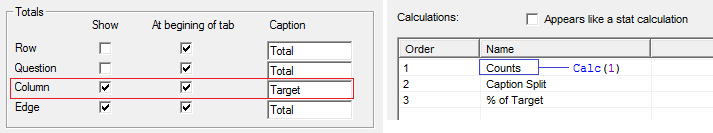The Size of arrTarget is 2 in this case for all the response captions (because they have one pipe delimiter and therefore a result either side of it when we split by pipe). The reason to use size in this case rather than hard code 2 is there is a possibility your delimiter is used in the response text (more likely if using ‘-‘ or ‘/’ as delimiters), therefore using size will always ensure last split section will be used (the correct one containing the target).

So when we are on the ‘Target’ column we return the target value obtained from the caption, otherwise we return Calc(1) – the counts stored in the variable.

% of Target:

`Dim arrTarget = currenttable.getcell(1,currentcell.y-1).Text.Split("|")return On(CurrentCell.column.index < 1,100,(Calc(1)/arrTarget[Size(arrTarget)].ToNumber())*100)`

The % of Target calculation is one cell below Caption Split so in order to access the arrTarget again we simply use currentcell.y-1 instead of currentcell.y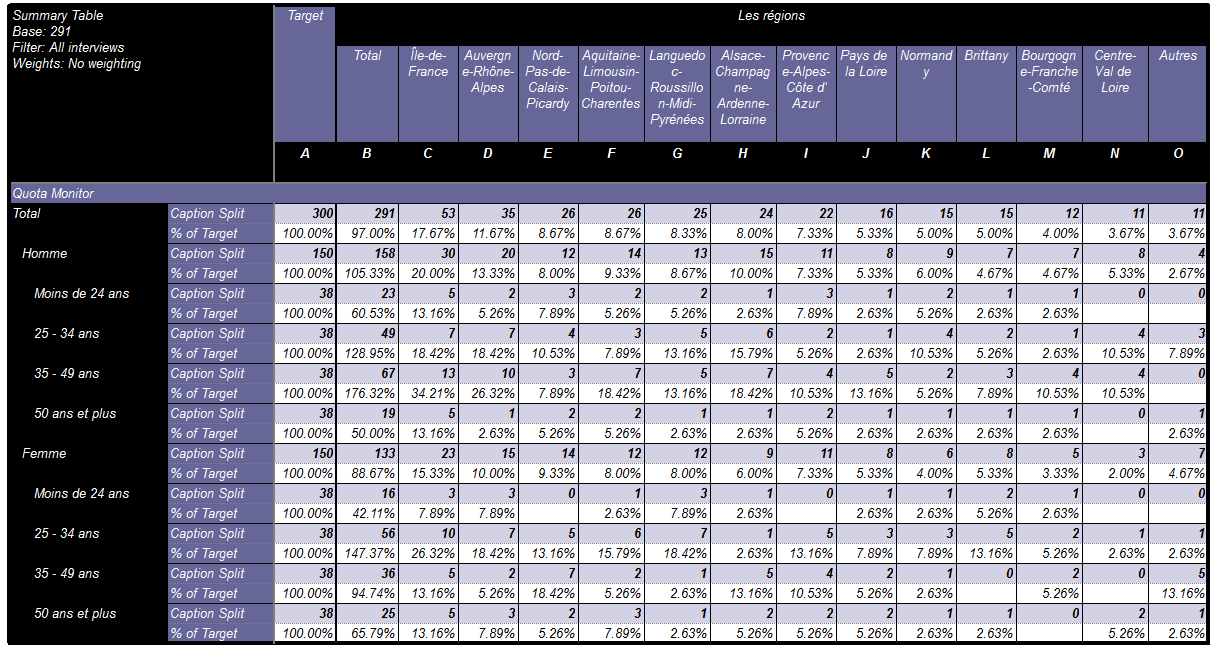The % achieved will always be 100 when in the Target column. When we are in the other columns, however, we perform the calculation:

`(Calc(1)/arrTarget[Size(arrTarget)].ToNumber())*100  i.e.    ( Counts   /  Target )  *  100`

Finally, we add some cleaning script to replace the response captions in the first column with:

`Dim jFor j = CurrentTable.StartY to CurrentTable.MaxYDim arrTarget = currenttable.getcell(1,j).Text.Split("|")currenttable.getcell(1,j).SetText(arrTarget)Next j`

Example 3

The third example uses ‘[Ex3] Crossed / Calc Arithmetic & Cleaning Script’  and is for a similar table layout. Rather than using the

`currenttable.getcell(1,currentcell.y).Text`

to access the text of the first column in the table, it uses:

`currentcell.row.question.responses[rIndex].caption`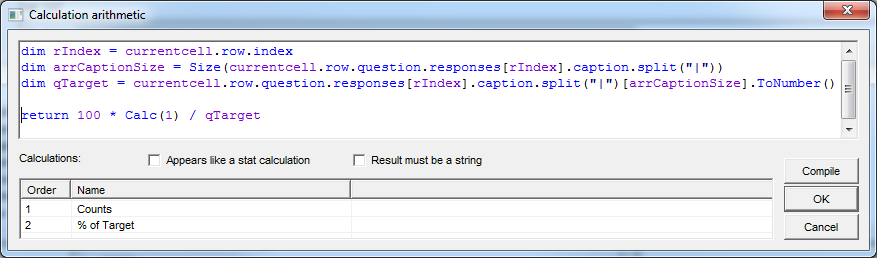Why is it favourable to use a reference to the row (Example 3) rather than the current cell (Example 2)? Well, if you intend to add calculations in between the Caption Split and % of Target calculations then the script from Example 2 will no longer work unless you update the syntax in both calculations. Adding calculations in Example 3 does not cause such an issue.

However, it’s worth noting two points here:

• grouped responses in the rows will cause currentcell.row.question to not count in sequence of selected responses (i.e. the deselected codes will also be counted if you use 'Group and hide' or 'Group and leave')
• calculated or grouped responses will not be considered (as a response) when using currentcell.row.question.responses - the response property of the question lists responses from its source structure

–  This is easy to work around by putting in place a created variable which does the same as the grouped response – see Quota Monitor 2 variable. In case the calculated response cannot be replicated in a created variable then you can revert to currenttable.getcell("...") syntax as shown in Example 2.

The cleaning script to be applied in this example:

`Dim jFor j = CurrentTable.StartY to CurrentTable.MaxY  Dim arrTarget = currenttable.getcell(1,j).Text.Split("|")  currenttable.getcell(1,j).SetText(arrTarget)  currenttable.getcell(3,j).SetText(arrTarget[size(arrTarget)])Next j`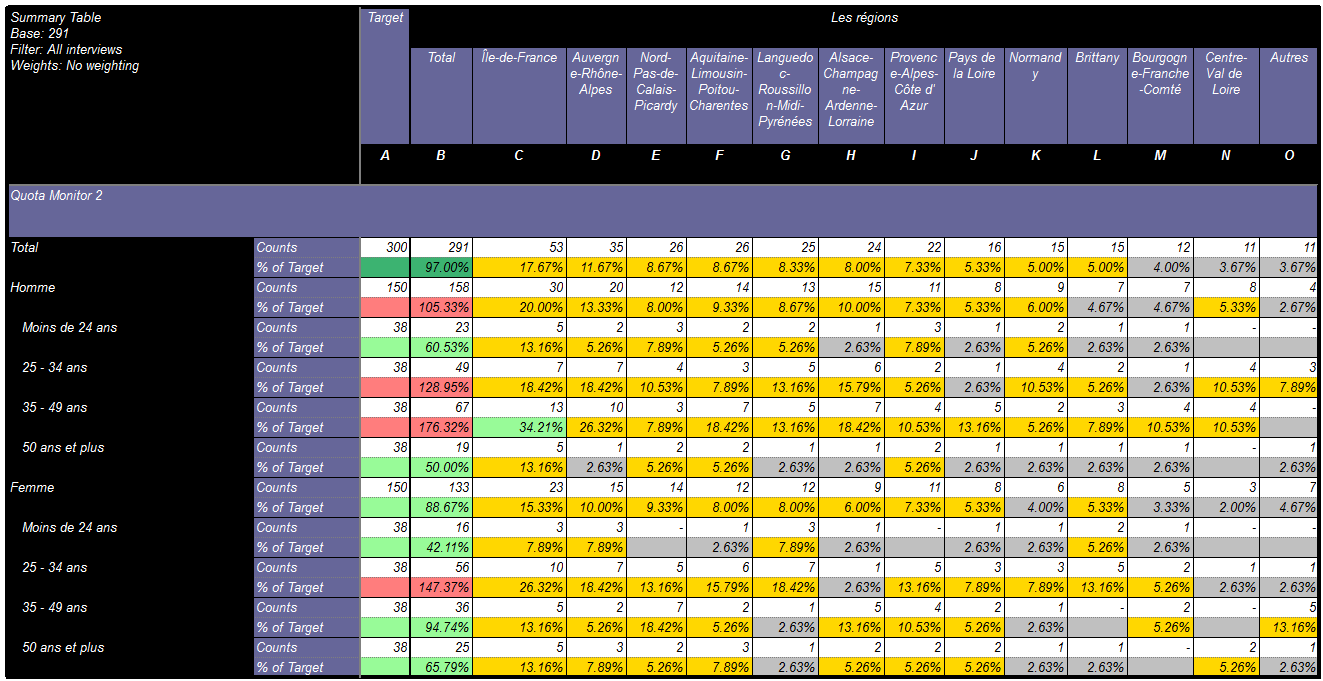Set up in Vista

So how do we get this in Vista?

• Create the survey in Vista as normal – it must have the calculated Quota variable that has been created in Analyse
• Recreate the tab definition and calculation as set out in Analyse
• Add your scripts from the Analyse calculations in to the same in Vista – note that currently Vista treats the captions differently and in order to give the same result we need to add some syntax:

Text.Replace("{\"Caption\":\"","").Replace("\"}","").Split("|")

Caption.Replace("{\"Caption\":\"","").Replace("\"}","").Split("|")

Here is the portfolio link for the examples discussed: